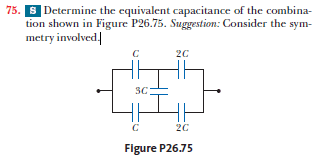# Equivalent Capacitance of Complex Circuit

## Homework Statement

Determine the equivalent capacitance of the combination
shown in Figure P26.75. Suggestion: Consider the symmetry
involved.## Homework Equations

Parallel is: C1+C2+C3+...
Series is: (C1^-1 + C2^-1 + C3^-1 + ...)^-1
q = CV

## The Attempt at a Solution

I have no idea how to do it for a complex circuit. I think it might have something to do with Kirchhoff's Law, but the book never mentioned it and neither did the professor, so I'm thinking it can do it without. And I am not sure what "the symmetry involved" is supposed to mean.

ehild
Homework Helper
Do you see any change if you turn the figure upside down?
Is there any reason that both "C" capacitors are different or the both 2C capacitors are?

If they are equivalent, so is the charge and voltage across them.What is the voltage across the 3C capacitor then?

ehild

ehild, thank you so much! Since the voltage across it is zero, it's like the 3C capacitor isn't there, right?

Solving the simple circuit then gives 4/3 C, which is the correct answer. Is my way of solving it correct? Or did I simply get the right answer the wrong way?

Many thanks!

Also, this isn't a problem I have to do, but what if the circuit were asymmetrical? Then what would you do?

ehild
Homework Helper

It is a very useful trick for symmetric circuits, that we can connect those points which are at the same potential with a single wire, so the points become a single node. Symmetrically equivalent points are at the same potential.

In general problems, you need to apply Kirchhoff's voltage Law and also the equivalent of Current Law, but with charges.

Every capacitor has its own charge, q at one plate and -q on the other plate.
The net charge at a node is zero. When the capacitors are connected in series, they all have the same charge.
And you know the relation between charge and voltage: q=CV.

Set up all equations and solve.

ehild

can you do Kirchoff's Law for this problem? How would you take into account the initial part before the loops and the last wire after the loops?

ehild
Homework Helper
Connect a voltage source to the terminals with arbitrary emf E. Write up the equations in term of E, and solve for the whole charge Q on the connected plates.
Te circuit can be représented by an equivalent capacitor connected to the same voltage source and you can apply C(equivalent)=Q/E

ehild